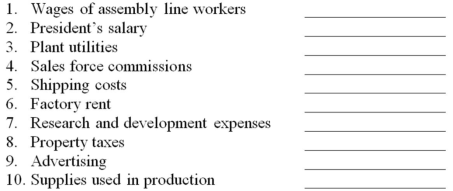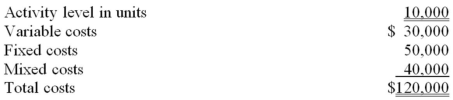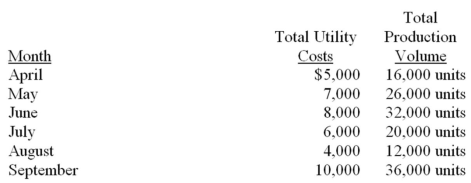• FAQ
• Contact/ Homework Answers / Accounting / 51.The contribution margin ratio always decreases when the:   A. break-even point decreases. B. fixed
Not my Question
Flag Content

# Question

51.The contribution margin ratio always decreases when the:

A. break-even point decreases.

B. fixed expenses increase.

C. selling price increases and the variable costs remain constant.

D. variable cost increase and the selling price remains constant.

52.If fixed costs were increased by \$9,000 and the contribution margin ratio remained at 30 percent, then sales must increase by _________ in order to cover the additional fixed expenses.

A. \$27,000

B. \$30,000

C. \$33,000

D. \$54,000

53.ABU Co. has several products, each with a different contribution margin ratio. If the same number of units were sold in July as in June, but the sales mix changed:

A. operating income would be the same in June and July.

B. fixed expenses in July would be in a different relevant range than in June.

C. the company's overall contribution margin ratio would be the same in June and July.

D. total contribution margin in July would be different from that in June.

54.A firm's products have an average contribution margin ratio of 40%, which will be maintained for the next month even though fixed expenses are expected to rise by \$20,000. In order to keep operating income for the month from being affected, revenues will have to increase by:

A. \$8,000.

B. \$12,000.

C. \$20,000.

D. \$50,000.

55.Each of a company's two product lines has a different contribution margin ratio. If the company's total sales remain the same but the sales mix shifts toward selling more of the product with the higher contribution ratio, which of the following is true?

A. Operating income will increase.

B. The average contribution margin ratio will increase.

C. The break-even point will decrease.

D. All of these are true.

56.Each of a company's several product lines has a different contribution margin ratio. Total sales in 2014 were 20% higher than total sales in 2013. Total contribution margin for 2014 will be:

A. the same as it was in 2013, regardless of changes in sales mix.

B. 20% higher than it was in 2013, regardless of changes in sales mix.

C. more than 20% higher than it was in 2013, if the sales mix changes and proportionately more high contribution margin ratio products are sold in 2014 than in 2013.

D. less than 20% higher than it was in 2013, if the sales mix changes and proportionately more high contribution margin ratio products are sold in 2014 than in 2013.

Essay Questions

57.For each of the following costs, identify the cost behavior as variable, mixed, or fixed:58.XYZ Company incurred the following costs for the month of August when it observed an activity level of 10,000 units.During October, the activity level was 16,000 units, and the total costs incurred were \$150,000.

a. Calculate the variable costs, fixed costs, and mixed costs incurred during October.
b. Use the high-low method to calculate the cost formula for mixed costs.
c. If the activity level were expected to be 13,800 units for the month of December, what amount of total costs would be expected?

59.During the months of April through September, the following total utility costs were paid at various production volumes:a. Use the high-low method to calculate the cost formula utility costs.
b. If the production volume were expected to be 22,000 units for the month of November, what amount of total costs would be expected?

60.Preppy Co. makes and sells a single product. The current selling price is \$30 per unit. Variable costs are \$21 per unit, and fixed expenses total \$90,000 per month. Sales volume for July totaled 12,000 units.

a. Calculate the operating income for July.
b. Calculate the break-even point in units sold and total revenues.
c. Management is considering the use of automated production equipment. If this were done, variable costs would drop to \$15.00 per unit, but fixed expenses would increase to \$100,000 per month.

(1.) Calculate operating income at a volume of 12,000 units per month with the new cost structure.
(2.) Calculate the break-even point in units with the new cost structure.

12-1

## Solution 5 (1 Ratings )

Solved
Accounting 2 Months Ago 552 Views